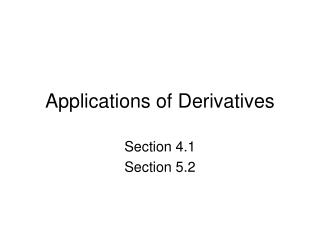Download PresentationApplications of Derivatives

# Applications of Derivatives - PowerPoint PPT PresentationDownload Presentation## Applications of Derivatives

- - - - - - - - - - - - - - - - - - - - - - - - - - - E N D - - - - - - - - - - - - - - - - - - - - - - - - - - -
##### Presentation Transcript

1. Applications of Derivatives Section 4.1 Section 5.2

2. Applications of Derivatives • Derivatives allow you to sketch the shape of functions

3. Applications of Derivatives • Ex: Amount of cargo unloaded at a port related to the number of trucks

4. Applications of Derivatives

5. Applications of Derivatives

6. Applications of Derivatives

7. Applications of Derivatives

8. Applications of Derivatives

9. Applications of Derivatives

10. Applications of Derivatives

11. Applications of Derivatives • Sketch the function c(w) based on the following: c(0) = 200 c(5) = 176 c(20) = 121 c’(0) = -50 c’(5) = -44 c’(20) = -30

12. Applications of Derivatives • Derivatives allow you to approximate functions

13. Applications of Derivatives

14. Applications of Derivatives

15. Applications of Derivatives

16. Applications of Derivatives

17. Applications of Derivatives

18. Applications of Derivatives

19. Applications of Derivatives

20. Applications of Derivatives • Suppose that for the function c(w), c(10) = 155 and c’(10) = -39. What is the approximate value of c(20)?

21. Extreme Points 0 + slope - slope

22. Extreme Points Population of Cleveland

23. Extreme Points

24. Extreme Points

25. Extreme Points

26. Extreme Points • Conclusions • At the minimum/maximum values of a function, the value of the derivative is 0. • At the inflection points of a function, the value of the derivative reaches a minimum/maximum.

27. Extreme Points • Finding roots • Easy for linear, quadratic • Hard for higher order polynomials, other function Y= GRAPH CALC 2: zero

28. Extreme Points • In-Class • Find the maxima and minima for the following functions • 0.04x3 - 0.88x2 + 4.81x +12.11 • 0.0004x4 – 0.007x3 + 0.03x2 – 0.035x + 10

29. Extreme Points • Cost of production • How many machines are needed to minimize the cost per unit?

30. Extreme Points

31. Extreme Points

32. Extreme Points • Fit a quadratic model to the data

33. Extreme Points • How many machines are needed to minimize the cost per unit?

34. Extreme Points

35. Extreme Points • How many machines are needed to minimize the cost per unit? • The number that sets c’(m) = 0 (root)

36. Extreme Points • Revenue over time • In what month was revenue maximized?

37. Extreme Points

38. Extreme Points

39. Extreme Points • Fit a quartic model to the data

40. Extreme Points • In what month was revenue maximized?

41. Extreme Points

42. Extreme Points • In what month was revenue maximized? • Find the 3 numbers that set r’(t) = 0 Y= GRAPH CALC 2: zero

43. Extreme Points • In what month was revenue maximized? • Find the 3 numbers that set r’(t) = 0

44. Extreme Points

45. Extreme Points • In-Class